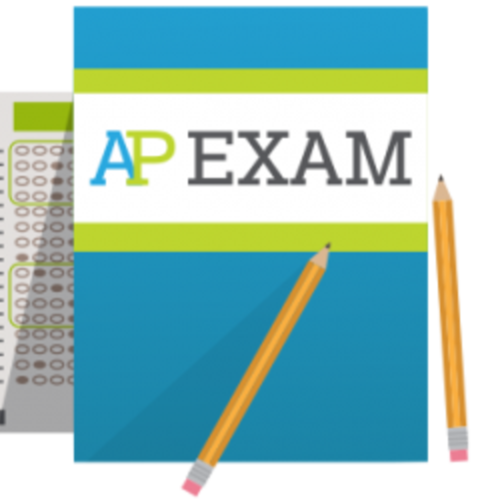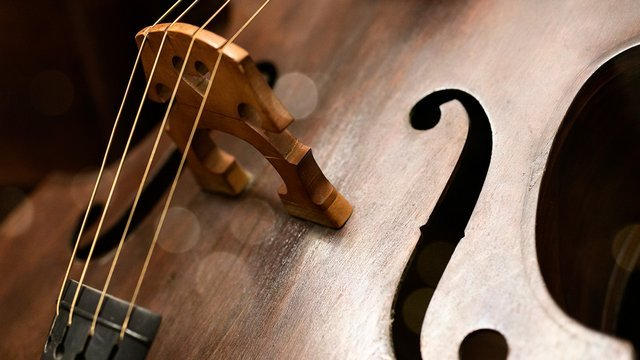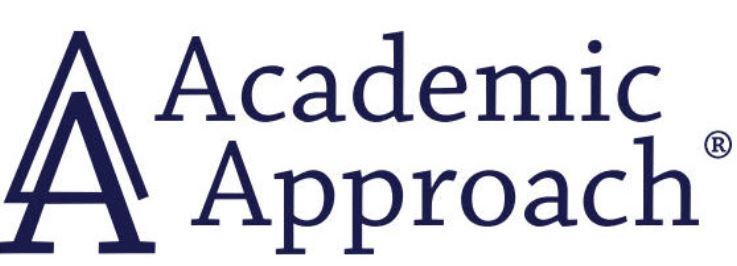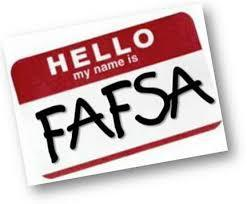# Upcoming Important Dates

Thursday, December 17 - Cozy Winter Virtual Showcase: Orchestra Concert
Saturday, December 19 - AP Payment Deadline
Monday, December 21 - Friday, January 1 - Winter Break

 table div table+table+table+table div table{width:100%;padding:0}table div table+table+table+table div table img{width:96.23%;padding:0;float:none}table div table+table+table+table div table td{width:100%;padding:0 1.88% 18px}/* styles */▪ Annual Appeal Update
▪ AP Exams and Payment
▪ Cozy Winter Virtual Showcase: Intermediate Strings, Concert Strings, Chamber Strings
▪ Winter Break
▪ The College Crisis Initiative: Panel Discussion at Davidson College This Wednesday, December 9
▪ Academic Approach SAT Test Prep
▪ College Application and Essay Resources
▪ College Representative Visits
▪ Financial Aid Resources
▪ FON Senior Parent College Night Highlights
▪ Photo Submissions Requested for NCP Stampede!
▪ Order Your 2020-2021 NCP Yearbook
▪ Ways for Parents to Stay in Touch
 ▪ Annual Appeal Update
 ▪ AP Exams and Payment
 ▪ Cozy Winter Virtual Showcase: Intermediate Strings, Concert Strings, Chamber Strings
 ▪ Winter Break
 ▪ The College Crisis Initiative: Panel Discussion at Davidson College This Wednesday, December 9
 ▪ Academic Approach SAT Test Prep
 ▪ College Application and Essay Resources
 ▪ College Representative Visits
 ▪ Financial Aid Resources
 ▪ FON Senior Parent College Night Highlights
 ▪ Photo Submissions Requested for NCP Stampede!
 ▪ Order Your 2020-2021 NCP Yearbook
 ▪ Ways for Parents to Stay in Touch
 table div table+table+table+table+table+table div table{width:100%;padding:0}table div table+table+table+table+table+table div table img{width:96.23%;padding:0;float:none}table div table+table+table+table+table+table div table td{width:100%;padding:0 1.88% 18px}/* styles */# Update on the 2020-2021 Annual Appeal

Thank you to all the families that have contributed thus far to the Annual Appeal!

Here is where our donation totals stand compared to prior years immediately after Giving Tuesday:

▪ 2020-2021 - \$75,000
▪ 2019-2020 - \$54,000
▪ 2018-2019 - \$100,000
▪ 2017-2018 - \$109,000
▪ 2016-2017 - \$99,000
 ▪ 2020-2021 - \$75,000
 ▪ 2019-2020 - \$54,000
 ▪ 2018-2019 - \$100,000
 ▪ 2017-2018 - \$109,000
 ▪ 2016-2017 - \$99,000

Contributions are ahead of last year, which is great. However, we are still behind contribution levels in the prior three years following Giving Tuesday.

Every dollar matters as they fund activities important to our students and which are not covered by the budget of Chicago Public Schools. Northside is annually ranked one of the top schools both in Illinois and the United States by U.S. News and World Report. Donations to Friends of Northside help fund activities to keep Northside the great school it is.

## Employer Donations

Please check with your employer about company matching donations. It can often double your support to Northside. Contact Friends of Northside at info@friendsofnorthside.org with any questions.

## Stock Donations

Friends of Northside graciously accepts donations of stock, which we convert to cash upon receipt. Please contact us at info@friendsofnorthside.org. FON can easily provide information related to Wintrust Bank if you would like to donate stock to FON in lieu of cash.

## PayPal

New this year is the ability to donate via PayPal. If you might normally write a check but now instead use PayPal, we encourage you to make online donations in this manner.

 table div table+table+table+table+table+table+table+table+table+table div table{width:100%;padding:0}table div table+table+table+table+table+table+table+table+table+table div table img{width:96.23%;padding:0;float:none}table div table+table+table+table+table+table+table+table+table+table div table td{width:100%;padding:0 1.88% 18px}/* styles */# AP Exams and Payment

There continues to be a technical issue with the collection of AP Exam fees. In prior communication, AP Exam fee payments would be due by Friday, December 4 at 3:00 pm. The payment deadline has been adjusted and will be later in December.

An announcement will be made to all students, teachers, and families to ensure that you are able to pay on time. Your continued patience is very much appreciated.

AP Exams will take place May 3-7 and May 10-14. For reference, the AP exam cost is \$110 per exam. If you completed your family income form and have been approved, the exam cost is \$15 per exam. For students enrolled in AP Seminar and Research, the exam cost is \$143.

 table div table+table+table+table+table+table+table+table+table+table+table+table div table{width:100%;padding:0}table div table+table+table+table+table+table+table+table+table+table+table+table div table img{width:96.23%;padding:0;float:none}table div table+table+table+table+table+table+table+table+table+table+table+table div table td{width:100%;padding:0 1.88% 18px}/* styles */# Cozy Winter Virtual Showcase

Thursday, December 17
7:00 pm

(Intermediate Strings, Concert Strings, Chamber Strings)

 table div table+table+table+table+table+table+table+table+table+table+table+table+table+table div table{width:100%;padding:0}table div table+table+table+table+table+table+table+table+table+table+table+table+table+table div table img{width:96.23%;padding:0;float:none}table div table+table+table+table+table+table+table+table+table+table+table+table+table+table div table td{width:100%;padding:0 1.88% 18px}/* styles */# Winter Break

Monday, December 21, 2020 - Friday, January 1, 2021

*Classes resume on Monday, January 4, 2021.

 table div table+table+table+table+table+table+table+table+table+table+table+table+table+table+table+table div table{width:100%;padding:0}table div table+table+table+table+table+table+table+table+table+table+table+table+table+table+table+table div table img{width:96.23%;padding:0;float:none}table div table+table+table+table+table+table+table+table+table+table+table+table+table+table+table+table div table td{width:100%;padding:0 1.88% 18px}/* styles */# Any Questions About NCP Communications Sent Via CNXT?

If you are having problems with SchoolCNXT, please contact the main office from 7:30 am - 3:15 pm at 773.534.3954, or you can email the NCP school clerk, Mary Grasz, at mtgrasz@cpu.edu.

NCP uses SchoolCNXT to communicate important information to parents and the school community. All families should have received a text message and/or email communication about signing up. If you did not receive that communication or need access to your student's account, you can gain access by using this tool on Northside's website.

 table div table+table+table+table+table+table+table+table+table+table+table+table+table+table+table+table+table+table+table div table{width:100%;padding:0}table div table+table+table+table+table+table+table+table+table+table+table+table+table+table+table+table+table+table+table div table img{width:96.23%;padding:0;float:none}table div table+table+table+table+table+table+table+table+table+table+table+table+table+table+table+table+table+table+table div table td{width:100%;padding:0 1.88% 18px}/* styles */# The College Crisis Initiative: Panel Discussion at Davidson College This Wednesday, December 9

As the semester draws to a close, we hope you will join us for a panel discussion at Davidson College that highlights an incredible example of the convergence of collaborative research, the liberal arts, and impact.

Born out of the COVID-19 global pandemic, The College Crisis Initiative (C2i) focuses on how colleges and universities innovate in a crisis mindset.

Since March, the C2i team at Davidson, comprised of student researchers and faculty, has collected and analyzed data on how post-secondary institutions have responded to the pandemic. The team shares their work with policymakers and the public via the C2i Dashboard.

Join us on December 9 at 8:00 pm EDT (7:00 pm CST) to learn about the genesis of the initiative, what the data is telling us, and what's next. Register now!

 table div table+table+table+table+table+table+table+table+table+table+table+table+table+table+table+table+table+table+table+table+table div table{width:100%;padding:0}table div table+table+table+table+table+table+table+table+table+table+table+table+table+table+table+table+table+table+table+table+table div table img{width:96.23%;padding:0;float:none}table div table+table+table+table+table+table+table+table+table+table+table+table+table+table+table+table+table+table+table+table+table div table td{width:100%;padding:0 1.88% 18px}/* styles */# Academic Approach SAT Test Prep

Academic Approach is offering an optional virtual SAT prep course to help NCP juniors and sophomores prepare for the Spring SAT School Day test scheduled for April 2021. It runs from January 20th-March 24, 2021 to help students prepare for the spring SAT in April. Information regarding the schedule, registration details, and course fee is available by following the link below. The deadline to register is December 27, 2020. Click here for more details.

 table div table+table+table+table+table+table+table+table+table+table+table+table+table+table+table+table+table+table+table+table+table+table+table div table{width:100%;padding:0}table div table+table+table+table+table+table+table+table+table+table+table+table+table+table+table+table+table+table+table+table+table+table+table div table img{width:96.23%;padding:0;float:none}table div table+table+table+table+table+table+table+table+table+table+table+table+table+table+table+table+table+table+table+table+table+table+table div table td{width:100%;padding:0 1.88% 18px}/* styles */# College Application and Essay Resources

Hello, Class of 2021 Parents,

Time is moving at light speed and the December college application deadlines are quickly approaching. New York University has you covered with helpful tips and best practices as your students prepare to hit submit on their college applications!

Here are a few articles that will assist you in supporting your students as they tackle their college applications and essays.

If you have any questions or concerns, do not hesitate to contact Ms. Moore at mcmoore6@cps.edu or email your student’s respective school counselor. We are here to support your family with navigating the next steps in the college admissions process.

▪ 3 Tips to Choose the Right Common App Essay Prompt (click here)
▪ What I Wish I Knew Before Applying to College (click here)

 table div table+table+table+table+table+table+table+table+table+table+table+table+table+table+table+table+table+table+table+table+table+table+table+table+table+table div table{width:100%;padding:0}table div table+table+table+table+table+table+table+table+table+table+table+table+table+table+table+table+table+table+table+table+table+table+table+table+table+table div table img{width:96.23%;padding:0;float:none}table div table+table+table+table+table+table+table+table+table+table+table+table+table+table+table+table+table+table+table+table+table+table+table+table+table+table div table td{width:100%;padding:0 1.88% 18px}/* styles */# College Representative Visits

The NCP Counseling Department is offering virtual postsecondary visits for Northside Prep students and parents. We are offering virtual postsecondary visits to ensure all families have the opportunity to explore various postsecondary pathways, engage with the postsecondary representatives, and obtain answers to pertinent questions or concerns. Virtual postsecondary visits are offered after school from 3:15 pm - 4:00 pm and during Flex Blocks 1-4 on Wednesdays.

To date, college, military, and GAP Year representatives are scheduled to connect with NCP students virtually, and the list is growing every day. Click HERE to view the list of upcoming and past postsecondary visits. Also, check out the messages from the admissions representatives and the additional resources they shared during their visits.

*Please note, all virtual visits will be conducted via CPS Google Meets and no email addresses outside CPS will be permitted into the virtual postsecondary visits. To protect the privacy and safety of students, parents will only be permitted to access virtual visits if their respective student is present and the student will be required to log in via their CPS Gmail account. All postsecondary visits will be managed and monitored by an NCP staff member.

 table div table+table+table+table+table+table+table+table+table+table+table+table+table+table+table+table+table+table+table+table+table+table+table+table+table+table+table+table div table{width:100%;padding:0}table div table+table+table+table+table+table+table+table+table+table+table+table+table+table+table+table+table+table+table+table+table+table+table+table+table+table+table+table div table img{width:96.23%;padding:0;float:none}table div table+table+table+table+table+table+table+table+table+table+table+table+table+table+table+table+table+table+table+table+table+table+table+table+table+table+table+table div table td{width:100%;padding:0 1.88% 18px}/* styles */# Financial Aid Resources

Video: Q&A with the Director of Financial Aid at Notre Dame (click here): Director of Financial Aid Mary Nucciarone answered questions from students and families during a recent University of Notre Dame Admissions LIVE event. Watch the session on-demand for insights and information about the financial aid process.

How Personal Savings are Considered on the FAFSA (click here): Many families think that saving for college may hurt their chances of getting financial aid. The reality is that the money the average family saves will not significantly affect the amount of aid they receive. There are several reasons why.

Financial Aid Q&A: Take this Chart to Your Next College Visit (click here): This handy chart lists 10 common financial aid questions. Ask them at your college visits and fill in the answers to get a full picture of the financial aid processes at your top-choice schools. We've included our answers too.

Presentations

Net Price Calculators

## Update: Recursos de presentación en Español

▪ Solicitud Alternativa para aplicar para la Subvención MAP de Illinois 2020-2021 (click here)
 ▪ Solicitud Alternativa para aplicar para la Subvención MAP de Illinois 2020-2021 (click here)
 table div table+table+table+table+table+table+table+table+table+table+table+table+table+table+table+table+table+table+table+table+table+table+table+table+table+table+table+table+table+table+table div table{width:100%;padding:0}table div table+table+table+table+table+table+table+table+table+table+table+table+table+table+table+table+table+table+table+table+table+table+table+table+table+table+table+table+table+table+table div table img{width:96.23%;padding:0;float:none}table div table+table+table+table+table+table+table+table+table+table+table+table+table+table+table+table+table+table+table+table+table+table+table+table+table+table+table+table+table+table+table div table td{width:100%;padding:0 1.88% 18px}/* styles */# CPS Scholarship Notifications and College Events Around the City

For more details about upcoming events and to access the most recent updates, (click HERE) to view the College Calendar on the Northside Prep Counseling Website. Also, for additional scholarship opportunities visit the Northside College Prep Counseling page (click HERE).

Monday, December 14
App Closes: The Science Ambassador Scholarship

Tuesday, December 15
App Closes: Golden Apple Scholars of Illinois

Thursday, December 31
App Closes: Gen and Kelly Tanabe Scholarship

Saturday, January 2
App Closes: Dolores Saxton Walker Scholarship

 table div table+table+table+table+table+table+table+table+table+table+table+table+table+table+table+table+table+table+table+table+table+table+table+table+table+table+table+table+table+table+table+table+table div table{width:100%;padding:0}table div table+table+table+table+table+table+table+table+table+table+table+table+table+table+table+table+table+table+table+table+table+table+table+table+table+table+table+table+table+table+table+table+table div table img{width:96.23%;padding:0;float:none}table div table+table+table+table+table+table+table+table+table+table+table+table+table+table+table+table+table+table+table+table+table+table+table+table+table+table+table+table+table+table+table+table+table div table td{width:100%;padding:0 1.88% 18px}/* styles */# FON Senior Parent College Night Highlights

We have written up the highlights of FON’s Senior Parent College Night for all those who were not able to attend. Panelists discussed the college search and application process; financial aid; what virtual graduation was like; college orientation and finding a roommate; the decision of whether or not to attend college courses remotely; and other aspects of attending college during a pandemic. You can find the write-up HERE.

 table div table+table+table+table+table+table+table+table+table+table+table+table+table+table+table+table+table+table+table+table+table+table+table+table+table+table+table+table+table+table+table+table+table+table+table div table{width:100%;padding:0}table div table+table+table+table+table+table+table+table+table+table+table+table+table+table+table+table+table+table+table+table+table+table+table+table+table+table+table+table+table+table+table+table+table+table+table div table img{width:96.23%;padding:0;float:none}table div table+table+table+table+table+table+table+table+table+table+table+table+table+table+table+table+table+table+table+table+table+table+table+table+table+table+table+table+table+table+table+table+table+table+table div table td{width:100%;padding:0 1.88% 18px}/* styles */# Photo Submissions Requested for NCP Stampede!table div table+table+table+table+table+table+table+table+table+table+table+table+table+table+table+table+table+table+table+table+table+table+table+table+table+table+table+table+table+table+table+table+table+table+table+table+table+table div table{width:100%;padding:0}table div table+table+table+table+table+table+table+table+table+table+table+table+table+table+table+table+table+table+table+table+table+table+table+table+table+table+table+table+table+table+table+table+table+table+table+table+table+table div table img{width:96.23%;padding:0;float:none}table div table+table+table+table+table+table+table+table+table+table+table+table+table+table+table+table+table+table+table+table+table+table+table+table+table+table+table+table+table+table+table+table+table+table+table+table+table+table div table td{width:100%;padding:0 1.88% 18px}/* styles */# Order a Yearbook Today!

Copies are \$45 until March 1 and \$55 afterwards.

 table div table+table+table+table+table+table+table+table+table+table+table+table+table+table+table+table+table+table+table+table+table+table+table+table+table+table+table+table+table+table+table+table+table+table+table+table+table+table+table+table+table div table{width:100%;padding:0}table div table+table+table+table+table+table+table+table+table+table+table+table+table+table+table+table+table+table+table+table+table+table+table+table+table+table+table+table+table+table+table+table+table+table+table+table+table+table+table+table+table div table img{width:96.23%;padding:0;float:none}table div table+table+table+table+table+table+table+table+table+table+table+table+table+table+table+table+table+table+table+table+table+table+table+table+table+table+table+table+table+table+table+table+table+table+table+table+table+table+table+table+table div table td{width:100%;padding:0 1.88% 18px}/* styles */# Do you have news or team results to share with the Northside parent community?

 table div table+table+table+table+table+table+table+table+table+table+table+table+table+table+table+table+table+table+table+table+table+table+table+table+table+table+table+table+table+table+table+table+table+table+table+table+table+table+table+table+table+table+table+table div table{width:100%;padding:0}table div table+table+table+table+table+table+table+table+table+table+table+table+table+table+table+table+table+table+table+table+table+table+table+table+table+table+table+table+table+table+table+table+table+table+table+table+table+table+table+table+table+table+table+table div table img{width:96.23%;padding:0;float:none}table div table+table+table+table+table+table+table+table+table+table+table+table+table+table+table+table+table+table+table+table+table+table+table+table+table+table+table+table+table+table+table+table+table+table+table+table+table+table+table+table+table+table+table+table div table td{width:100%;padding:0 1.88% 18px}/* styles */# Support Northside - Shop AmazonSmile

AmazonSmile is a simple and automatic way to support Northside every time you shop.

Please note that our AmazonSmile name is Friends of "North" "Side" (North Side - two words at AmazonSmile).

 table div table+table+table+table+table+table+table+table+table+table+table+table+table+table+table+table+table+table+table+table+table+table+table+table+table+table+table+table+table+table+table+table+table+table+table+table+table+table+table+table+table+table+table+table+table+table div table{width:100%;padding:0}table div table+table+table+table+table+table+table+table+table+table+table+table+table+table+table+table+table+table+table+table+table+table+table+table+table+table+table+table+table+table+table+table+table+table+table+table+table+table+table+table+table+table+table+table+table+table div table img{width:96.23%;padding:0;float:none}table div table+table+table+table+table+table+table+table+table+table+table+table+table+table+table+table+table+table+table+table+table+table+table+table+table+table+table+table+table+table+table+table+table+table+table+table+table+table+table+table+table+table+table+table+table+table div table td{width:100%;padding:0 1.88% 18px}/* styles */# Follow Us on Social Media

 table div table+table+table+table+table+table+table+table+table+table+table+table+table+table+table+table+table+table+table+table+table+table+table+table+table+table+table+table+table+table+table+table+table+table+table+table+table+table+table+table+table+table+table+table+table+table+table+table div table{width:100%;padding:0}table div table+table+table+table+table+table+table+table+table+table+table+table+table+table+table+table+table+table+table+table+table+table+table+table+table+table+table+table+table+table+table+table+table+table+table+table+table+table+table+table+table+table+table+table+table+table+table+table div table img{width:96.23%;padding:0;float:none}table div table+table+table+table+table+table+table+table+table+table+table+table+table+table+table+table+table+table+table+table+table+table+table+table+table+table+table+table+table+table+table+table+table+table+table+table+table+table+table+table+table+table+table+table+table+table+table+table div table td{width:100%;padding:0 1.88% 18px}/* styles */table div table+table+table+table+table+table+table+table+table+table+table+table+table+table+table+table+table+table+table+table+table+table+table+table+table+table+table+table+table+table+table+table+table+table+table+table+table+table+table+table+table+table+table+table+table+table+table+table+table+table div table{width:100%;padding:0}table div table+table+table+table+table+table+table+table+table+table+table+table+table+table+table+table+table+table+table+table+table+table+table+table+table+table+table+table+table+table+table+table+table+table+table+table+table+table+table+table+table+table+table+table+table+table+table+table+table+table div table img{width:96.23%;padding:0;float:none}table div table+table+table+table+table+table+table+table+table+table+table+table+table+table+table+table+table+table+table+table+table+table+table+table+table+table+table+table+table+table+table+table+table+table+table+table+table+table+table+table+table+table+table+table+table+table+table+table+table+table div table td{width:100%;padding:0 1.88% 18px}/* styles */table div table+table+table+table+table+table+table+table+table+table+table+table+table+table+table+table+table+table+table+table+table+table+table+table+table+table+table+table+table+table+table+table+table+table+table+table+table+table+table+table+table+table+table+table+table+table+table+table+table+table+table+table+table div table{width:100%;padding:0}table div table+table+table+table+table+table+table+table+table+table+table+table+table+table+table+table+table+table+table+table+table+table+table+table+table+table+table+table+table+table+table+table+table+table+table+table+table+table+table+table+table+table+table+table+table+table+table+table+table+table+table+table+table div table img{width:96.23%;padding:0;float:none}table div table+table+table+table+table+table+table+table+table+table+table+table+table+table+table+table+table+table+table+table+table+table+table+table+table+table+table+table+table+table+table+table+table+table+table+table+table+table+table+table+table+table+table+table+table+table+table+table+table+table+table+table+table div table td{width:100%;padding:0 1.88% 18px}/* styles */